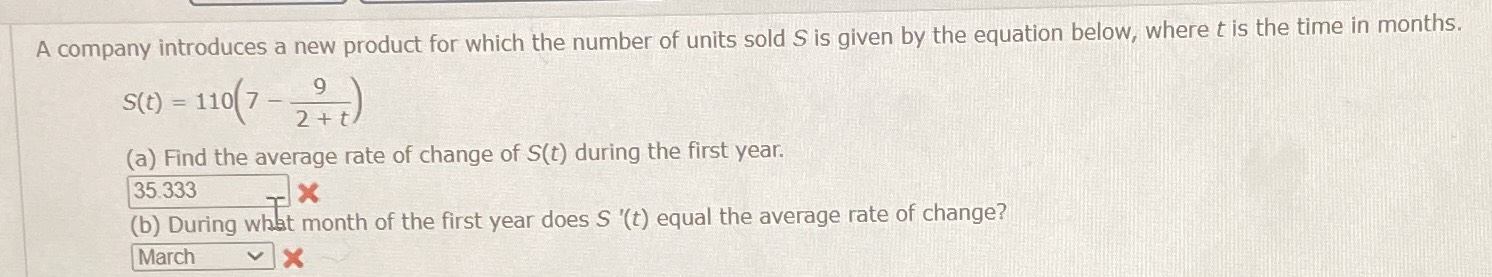### ¿Todavía tienes preguntas de matemáticas?

Pregunte a nuestros tutores expertos
Algebra
PreguntaA company introduces a new product for which the number of units sold $$S$$ is given by the equation below, where $$t$$ is the time in months.

$$S ( t ) = 110 ( 7 - \frac { 9 } { 2 + t } )$$

(a) Find the average rate of change of $$S ( t )$$ during the first year. $$\square$$

(b) During what month of the first year does $$S ^ { \prime } ( t )$$ equal the average rate of change? $$\square$$